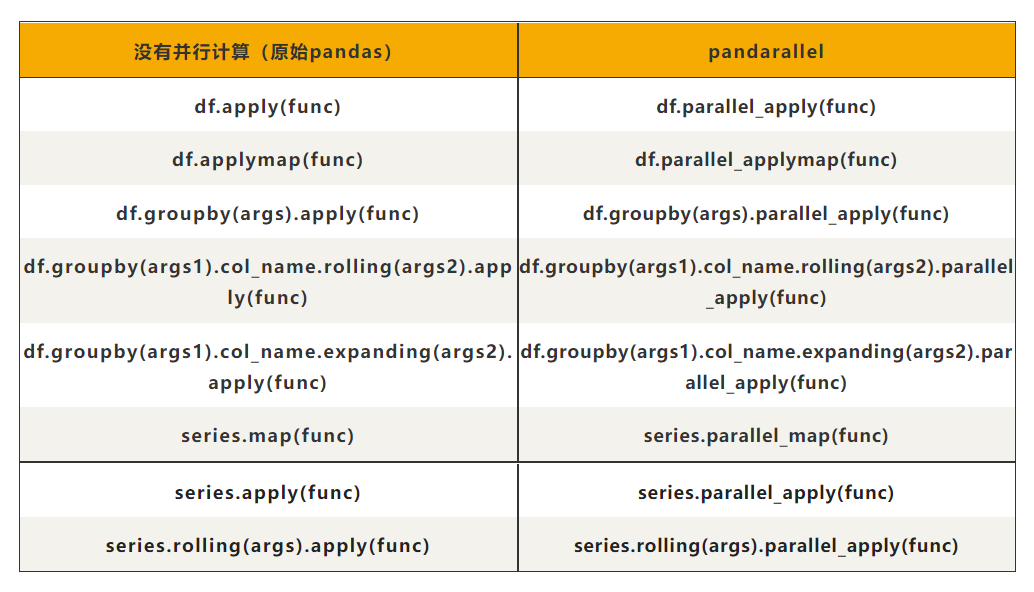﻿ Python提高运行速度工具之Pandarallel的使用教程_python_脚本之家
python# Python提高运行速度工具之Pandarallel的使用教程

• multiprocessing
• concurrent.futures.ProcessPoolExecutor()
• joblib
• ppserver
• celery## 1.准备

```pip install pandarallel
```

## 2.使用 Pandarallel

```from pandarallel import pandarallel
pandarallel.initialize()
```

Pandarallel一共支持8种Pandas操作，下面是一个apply方法的例子。

```import pandas as pd
import time
import math
import numpy as np
from pandarallel import pandarallel

# 初始化
pandarallel.initialize()
df_size = int(5e6)
df = pd.DataFrame(dict(a=np.random.randint(1, 8, df_size),
b=np.random.rand(df_size)))
def func(x):
return math.sin(x.a**2) + math.sin(x.b**2)

# 正常处理
res = df.apply(func, axis=1)

# 并行处理
res_parallel = df.parallel_apply(func, axis=1)

# 查看结果是否相同
res.equals(res_parallel)
```

```import pandas as pd
import time
import math
import numpy as np
from pandarallel import pandarallel

# 初始化
pandarallel.initialize()
df_size = int(3e7)
df = pd.DataFrame(dict(a=np.random.randint(1, 1000, df_size),
b=np.random.rand(df_size)))
def func(df):
dum = 0
for item in df.b:
dum += math.log10(math.sqrt(math.exp(item**2)))

return dum / len(df.b)

# 正常处理
res = df.groupby("a").apply(func)
# 并行处理
res_parallel = df.groupby("a").parallel_apply(func)
res.equals(res_parallel)
```

```import pandas as pd
import time
import math
import numpy as np
from pandarallel import pandarallel

# 初始化
pandarallel.initialize()
df_size = int(1e6)
df = pd.DataFrame(dict(a=np.random.randint(1, 300, df_size),
b=np.random.rand(df_size)))
def func(x):
return x.iloc + x.iloc ** 2 + x.iloc ** 3 + x.iloc ** 4

# 正常处理
res = df.groupby('a').b.rolling(4).apply(func, raw=False)
# 并行处理
res_parallel = df.groupby('a').b.rolling(4).parallel_apply(func, raw=False)
res.equals(res_parallel)
```## 3.注意事项

1. 我有 8 个 CPU，但 parallel_apply 只能加快大约4倍的计算速度。为什么？

2. 并行化是有成本的（实例化新进程，通过共享内存发送数据，…），所以只有当并行化的计算量足够大时，并行化才是有意义的。对于很少量的数据，使用 Pandarallel 并不总是值得的。